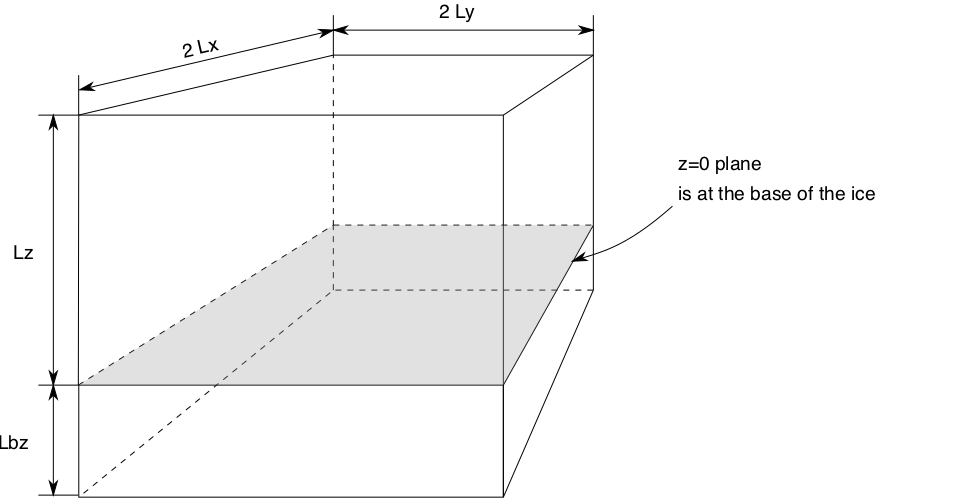# Computational box¶

PISM performs all simulations in a computational box which is rectangular in the PISM coordinates. The coordinate system has horizontal coordinates $$x,y$$ and a vertical coordinate $$z$$. The $$z$$ coordinate is measured positive upward from the base of the ice.1 The vector of gravity is in the negative $$z$$ direction. The surface $$z=0$$ is the base of the ice, however, and thus is usually not horizontal in the sense of being parallel to the geoid.

The surface $$z=0$$ is the base of the ice both when the ice is grounded and when the ice is floating.

When the ice is grounded, the true physical vertical coordinate $$z'$$, namely the coordinate measured relative to a reference geoid, is given by

$z' = z + b(x,y),$

where $$b(x,y)$$ is the bed topography. The top surface of the ice $$h(x,y)$$ is described by $$h(x,y) = H(x,y) + b(x,y)$$, where $$H(x,y)$$ is the ice thickness.

In the floating case, the physical vertical coordinate is

(1)$z' = z + z_{sl} - \frac{\rho_i}{\rho_w} H(x,y)$

where $$\rho_i$$ is the density of ice, $$\rho_w$$ the density of sea water, and $$z_{sl}$$ is the sea level elevation. Again, the physical elevation of the bottom (top) surface of the ice relative to the geoid can be computed by substituting $$z = 0$$ ($$z = H(x,y)$$) in (1).

Here the flotation criterion $$z_{sl} - \frac{\rho_i}{\rho_w} H(x,y) > b(x,y)$$ applies.

The computational box can extend downward into the bedrock. As $$z=0$$ is the base of the ice, the bedrock corresponds to negative $$z$$ values regardless of its true (i.e. $$z'$$) elevation.

The extent of the computational box, along with its bedrock extension downward, is determined by four numbers Lx, Ly, Lz, and Lbz (see Fig. 17 and Table 6). The first two of these are half-widths and have units of kilometers when set by command-line options or displayed.Fig. 17 PISM’s computational box

Table 6 Options defining the extent of PISM’s computational box

Option

Description

-Lx (km)

Half-width of the computational domain (in the $$x$$-direction)

-Ly (km)

Half-width of the computational domain (in the $$y$$-direction)

-Lz (meters)

Height of the computational domain; must exceed maximum ice thickness

-Lbz (meters)

Depth of the computational domain in the bedrock thermal layer

-x_range A,B (meters)

Specify the range of $$x$$ coordinates. Use this to select a subset of an input grid that isn’t in the center of a domain in PISM’s regional mode.

-y_range A,B (meters)

Specify the range of $$y$$ coordinates in PISM’s regional mode.

See Grid registration for details about the interpretation of $$L_x$$, $$L_y$$, and the way the grid spacing is computed.

Footnotes

1

 Previous Up Next